#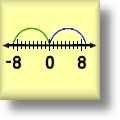Number Line Worksheets

## Dynamically Created Number Line Worksheets

Here is a graphic preview for all of the Dynamic Number Line Worksheets. You can select different variables to customize these Number Line Worksheets for your needs. The Number Line Worksheets are randomly created and will never repeat so you have an endless supply of quality Number Line Worksheets to use in the classroom or at home. We also produce blank Number Line Worksheets for your use. Our Number Line Worksheets are free to download, easy to use, and very flexible.

Click here for a Detailed Description of all the Number Line Worksheets.

## Quick Link for All Number Line Worksheets

Click the image to be taken to that Number Line Worksheet.

##### Horizontal Number LineGraphing Paper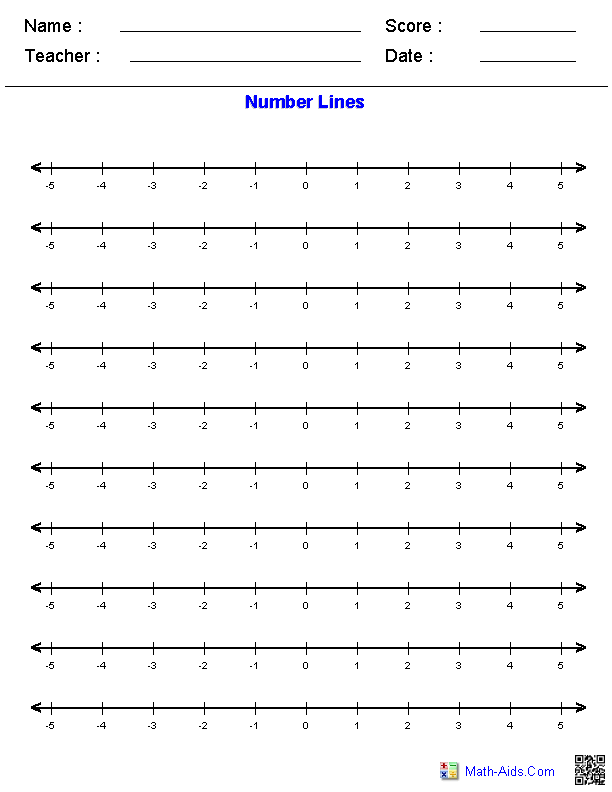##### Vertical Number LineGraphing Paper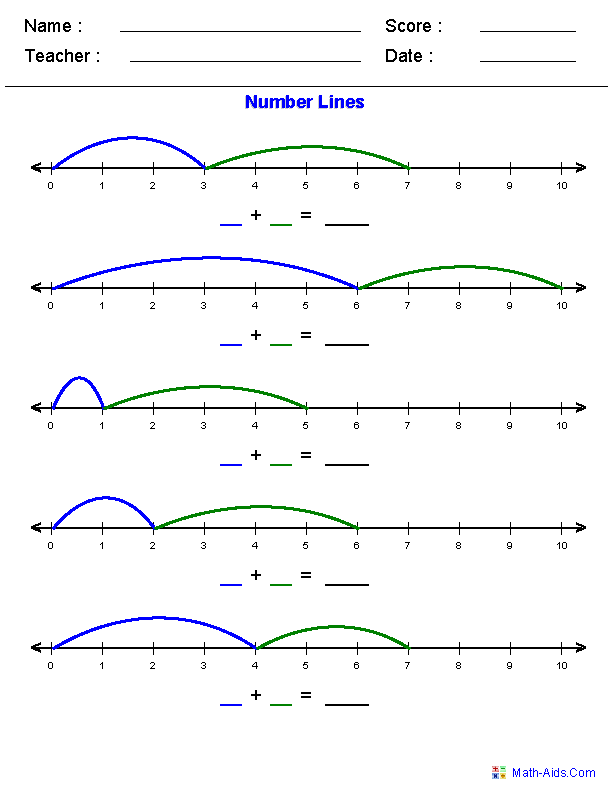##### Subtracting withNumber Lines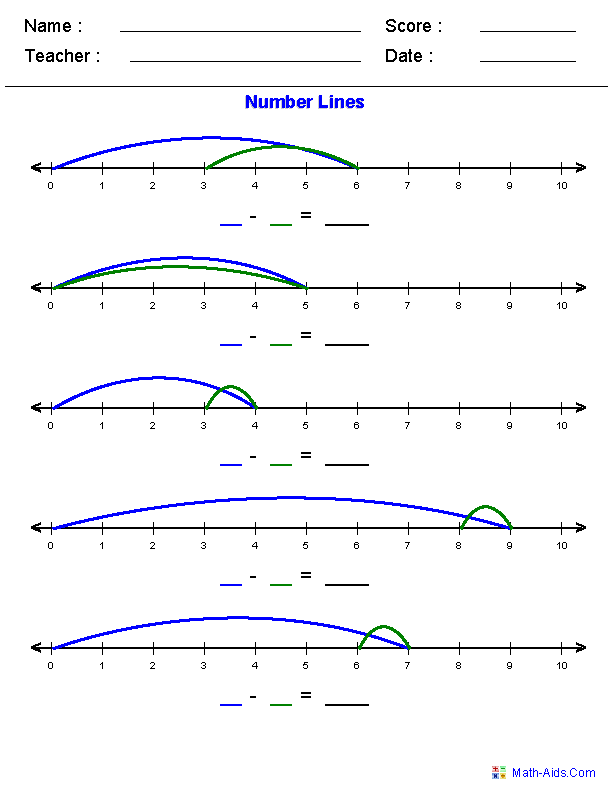##### Fractions onNumber Lines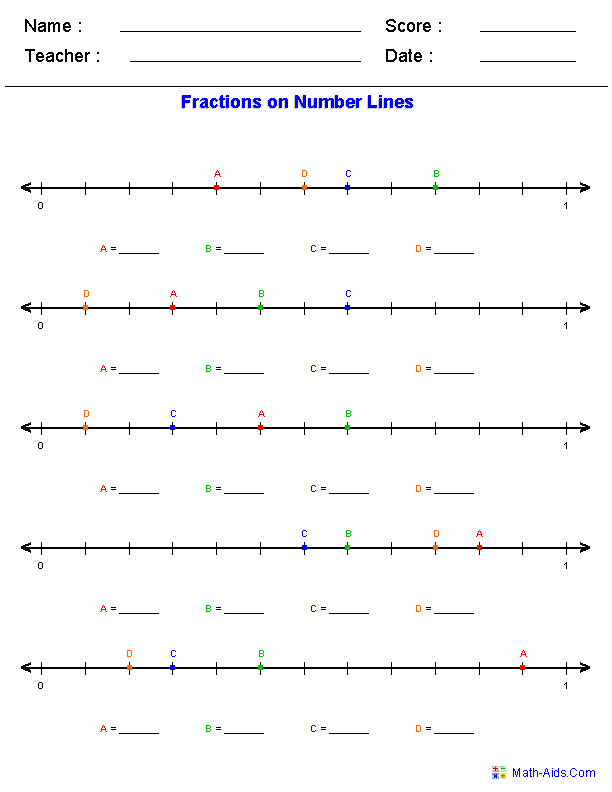##### Decimals onNumber Lines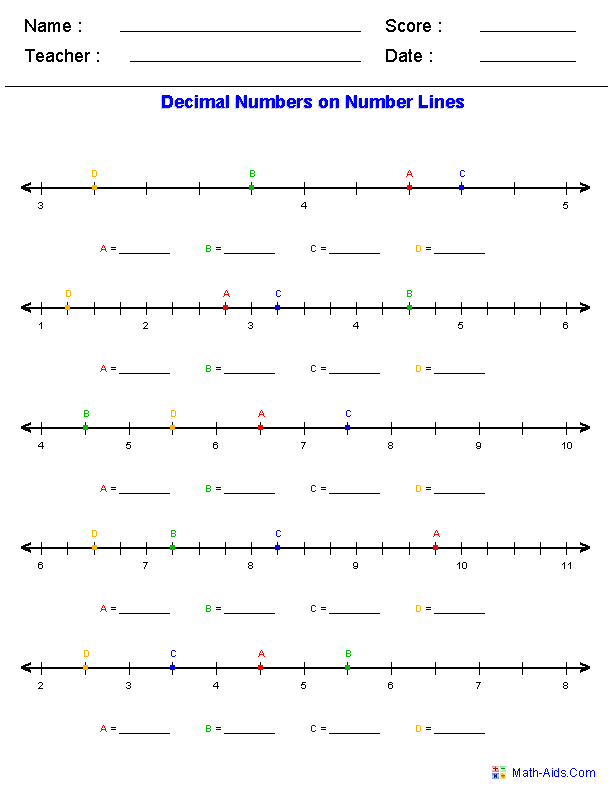##### Mixed Numbers onNumber Lines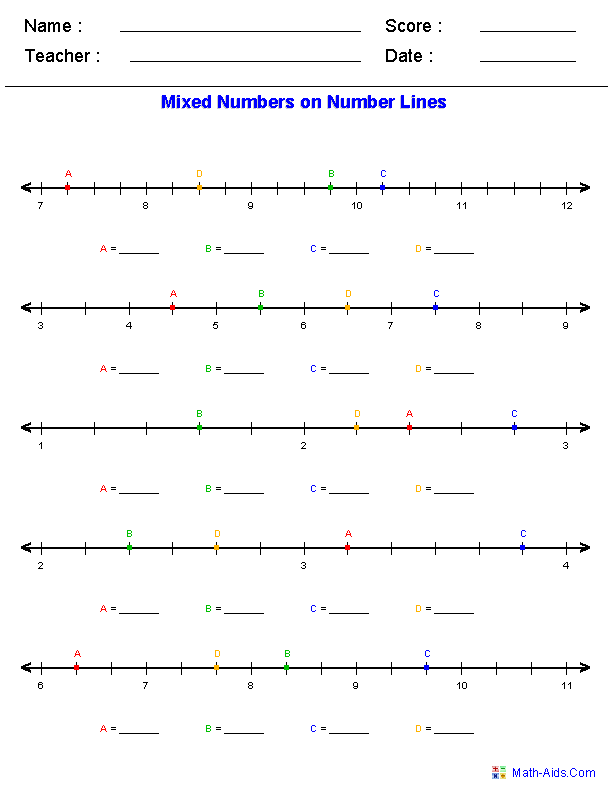Recommended Videos

## Detailed Description for All Number Line Worksheets

Horizontal Number Line Graphing Paper Worksheets
These Number Line Worksheets will produce a page of horizontal number lines for various types of scales, start points, end points and increments. These Number Line Worksheets are appropriate for Kindergarten, 1st Grade, 2nd Grade, 3rd Grade, 4th Grade, 5th Grade, and 6th Grade.

Vertical Number Line Graphing Paper Worksheets
These Number Line Worksheets will produce a page of vertical number lines for various types of scales, start points, end points and increments. These Number Line Worksheets are appropriate for Kindergarten, 1st Grade, 2nd Grade, 3rd Grade, 4th Grade, 5th Grade, and 6th Grade.

These Number Line Worksheets will produce addition number lines worksheets. You select different ranges and problem types. These Number Line Worksheets are appropriate for Kindergarten, 1st Grade, and 2nd Grade.

Subtracting with Number Lines Worksheets
These Number Line Worksheets will produce subtraction number lines worksheets. You select different ranges and problem types. These Number Line Worksheets are appropriate for Kindergarten, 1st Grade, and 2nd Grade.

Fractions on Number Lines Worksheets
These Number Line Worksheets will produce problems for the children to correctly mark the fractions on the given number lines. You may select positive or negative types of fractions for the problems.

Decimals on Number Lines Worksheets
These Number Line Worksheets will produce problems for the children to correctly mark the mixed numbers on the given number lines. You may select positive or negative decimals for the problems.

Mixed Numbers on Number Lines Worksheets
These Number Line Worksheets will produce problems for the children to correctly mark the mixed numbers on the given number lines. You may select positive or negative mixed numbers for the problems.